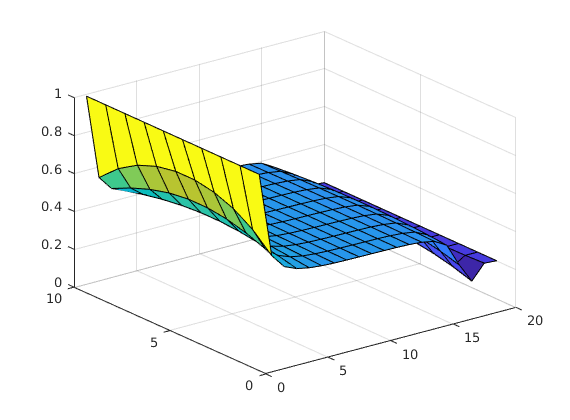# Tunel for Other Solvers

To start this tutorial, write the following command in the MATLAB console

open T044_TunelSolvers

## Tunel Solver Neos Server AMPL - IPOPT

Definition of the time %% Discretization of the space

N = 10;
L = 1;
xi = 0; xf = L;
xline = linspace(0,L,N+2);
xline = xline(2:end-1);
dx = xline(2) - xline(1);

Y = SymsVector('y',N);
U = SymsVector('u',1);


Diffusion part: the discretization of the 1d Laplacian

A = FDLaplacian(xline);
B = (N^2/L^2)*[1 ; zeros(N-2,1) ;1];

Fsym  = A*Y + B*U;
syms t
Fsym_fh = matlabFunction(Fsym,'Vars',{t,Y,U,sym.empty});


## Setting of equation

odeEqn = pde(Fsym_fh,Y,U);
odeEqn.InitialCondition = 0.99+0*xline';
odeEqn.Nt=20;
odeEqn.FinalTime = 2;
odeEqn.mesh{1} = xline;


We create the object that collects the formulation of an optimal control problem by means of the object that describes the dynamics odeEqn, the functional to minimize Jfun and the time horizon T

YT = 0.2 + 0*xline';
symPsi  = @(T,Y)   (YT - Y).'*(YT - Y);
symL    = @(t,Y,U) 0 ;
%% Create the pontryagin object
iCP1 = Pontryagin(odeEqn,symPsi,symL);
%% Define some constraints
iCP1.Constraints.MaxControl = 1;
iCP1.Constraints.MinControl = 0;


## Create a AMPL File from ICP1 object

AMPLFile(iCP1,'Domenec.txt')


## Send job of Neos Server

out = SendNeosServer('Domenec.txt');

Output in: /home/djoroya/Documentos/GitHub/DyCon-toolbox/tmp/AMPL-executions/27-Sep-2019-10-47-421076-054850-Domenec.txt/Domenec.txt.outdata = NeosLoadData(out);

figure;